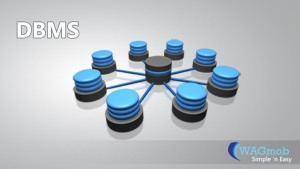41. What is normalization?
It is a process of analysing the given relation schemas based on their Functional Dependencies (FDs) and primary key to achieve the properties
(1).Minimizing redundancy, (2). Minimizing insertion, deletion and update anomalies.
42. What is Functional Dependency?
A Functional dependency is denoted by X Y between two sets of attributes X and Y that are subsets of R specifies a constraint on the possible tuple that can form a relation state r of R. The constraint is for any two tuples t1 and t2 in r if t1[X] = t2[X] then they have t1[Y] = t2[Y]. This means the value of X component of a tuple uniquely determines the value of component Y.

43. What is Lossless join property?
It guarantees that the spurious tuple generation does not occur with respect to relation schemas after decomposition.

44. What is 1 NF (Normal Form)?
The domain of attribute must include only atomic (simple, indivisible) values.

45. What is Fully Functional dependency?
It is based on concept of full functional dependency. A functional dependency X Y is full functional dependency if removal of any attribute A from X means that the dependency does not hold any more.

46. What is 2NF?

A relation schema R is in 2NF if it is in 1NF and every non-prime attribute A in R is fully functionally dependent on primary key.

47. What is 3NF?
A relation schema R is in 3NF if it is in 2NF and for every FD X A either of the following is true
X is a Super-key of R.
A is a prime attribute of R.
In other words, if every non prime attribute is non-transitively dependent on primary key.

48. What is BCNF (Boyce-Codd Normal Form)?
A relation schema R is in BCNF if it is in 3NF and satisfies an additional constraint that for every FD X A, X must be a candidate key.

49. What is 4NF?
A relation schema R is said to be in 4NF if for every Multivalued dependency X Y that holds over R, one of following is true.
1.) X is subset or equal to (or) XY = R.
2.) X is a super key.

50. What is 5NF?
A Relation schema R is said to be 5NF if for every join dependency {R1, R2, …, Rn} that holds R, one the following is true 1.) Ri = R for some i.
2.) The join dependency is implied by the set of FD, over R in which the left side is key of R.